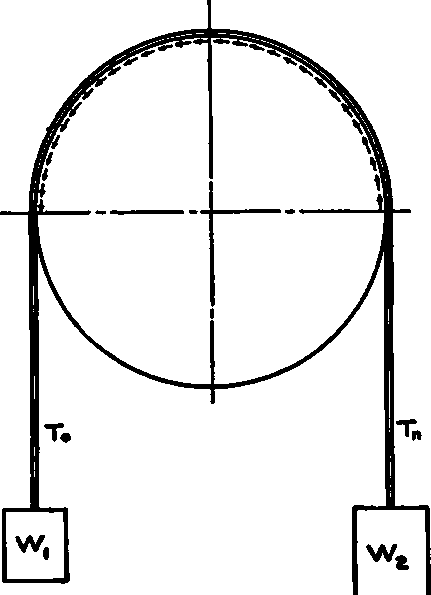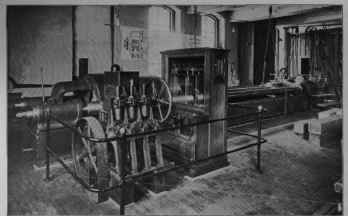### Theory

The condition of the tight and loose sides of a belt transmitting power, is similar to that of the weighted strap and fixed pulley shown in Fig. 17. If motion is desired of the strap around the pulley, it is necessary to make the weight W, of such a magnitude that it will overcome not only the weight W1 but also the friction between the strap and the pulley. The strap tension Tn is, of course, equal to W2, and T0 to W1. The equation showing the balance of forces for the condition when motion is about to occur, is:

Tn - To = F = P (driving force). (5)

If the pulley be free to turn on its axis, instead of being fixed as in Fig. 17, the strap by its friction on the pulley will turn the pulley, and the force of friction F becomes the driving force for the pulley as noted in equation 5 above.

In Fig. 18, let us suppose that W is a weight representing the resistance to be overcome. The tensions Tn and T0, equal at first owing to stretching the belt tightly over the pulleys at rest, change when an attempt is made to raise the weight by turning the larger pulley; and just as the weight leaves the floor, the equality of moments about the axis of the driven pulley gives the following equation:

(Tn-T0) r = F X r = P x r = W x r1. (6)

This equality of moments remains as long as the motion of the weight is uniform, and represents closely the conditions under which belt pulleys work.

Although we know from the above what the difference of the belt tensions is, and what this difference will do when applied to the surface of a given pulley, we do not yet know what either Tn or To actually is; and until we do know, we cannot correctly proportion the belt. Hence we must find another relation between Tn and To which we can combine with equations 5 and 6. This relation is deduced by a process of higher mathematics, which results as follows:Fig. 17.

Common logarithm Tn / To = 2.729 u (1-z) n . (7)

Treating equations 5 and 7 as simultaneous, values of both Tn and To can be found by the regular algebraic solution. As Tn is the larger, the actual area of belt to provide the necessary strength must be made to depend upon it.

The factor z in equation 7 depends upon the centrifugal force developed by the weight of the belt passing around the pulley. Its value, found from mechanics, is: z = w x V2 / 9,660 x t.Fig. 18.

Having found the maximum pull on the belt, it now remains to write the equation:

External force = Internal resistance; or, Tn = b X h X t. (8)

Usually the most convenient way to handle this equation is to assume h and t, and then solve for b.

Summing up the theoretical treatment of belt design, we simply combine equations 5, 6, 7, and 8, and solve for the quantity .desired. Discussion of the constants involved in these equations, and of the practical factors controlling them, is given in the following:

### Practical Modification

The force of friction F, which is the same as driving force P, depends on:

Coefficient of friction (u) between belt and pulley;

Tightness of the belt;

Centrifugal force of the belt;

Angle of contact of belt with pulley.

The coefficient of friction (u), according to experiments and observed operation of belts transmitting power, varies from .15 to .56 for leather on cast iron. An average value consistent with a reasonable amount of slip, the belt being in good running order, is .30. If the belt is oily, or likely to become so in use, a lower value should be taken.

The tighter the belt is drawn up, the greater is the pressure against the pulley, and hence the greater is the force of friction. But if we pull the belt up too tightly, when we begin to drive, Tn becomes too great, and the belt breaks or is under such stress that it wears out quickly. Moreover, the great side pressure on the bearings carrying the shaft produces excessive friction, and the drive is inefficient. This is why a narrow belt driven at high speed is more efficient than a wide belt at slow speed, for we can-not pull up the former as tightly as the latter without overstraining it, and yet it is possible to get the required power out of the narrow belt by running it at high speed.

The centrifugal force is of small importance for low speeds, say of 3,000 feet per minute and less; and it therefore may usually be neglected. The factor z then becomes zero in the expression 1 - z in equation 7, and the second member of the equation stands simply 2.729 X u X n.

The angle of contact of belt with pulley is important, as a large value gives a great difference between Tn and T0; and it is desirable to make this difference as great as possible, because thereby the driving force is increased. The loose side of a horizontal belt should always be above, as then the natural sag of the loose side due to its slackness tends to increase the angle of contact with the pulley, while the tightening up of the lower side acts against its sag to make the loss of wrap as little as possible. Vertical belts which have the driving pulley uppermost, utilize the weight of the belt to increase the pressure against the surface of the pulley, slightly increasing its capacity for driving. The angle of contact may be artificially increased by a tightening pulley which presses the belt further around the pulley than it would naturally lie. It adds however, the friction of its own bearing, and impairs the efficiency of the drive. For ordinary horizontal belts, the angle of contact is but little more than 180°, and the value of n in equation 7 may be safely assumed at ½ unless the pulleys are of relatively great difference of diameter and very close together.EMERY TESTING MACHINE. Mechancal Engineering Department, Massachusettsl Institute of Technology.